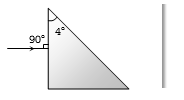# NEET Physics Ray Optics and Optical Instruments Questions SolvedSA prism having an apex angle 4o and refraction index 1.5 is located in front of a vertical plane mirror as shown in figure. Through what total angle is the ray deviated after reflection from the mirror(a)   176o

(b)   4o

(c)   178o

(d)   2o

(c)     ${S}_{prism}=\left(\mu -1\right)A=\left(1.5-1\right)4°=2°\phantom{\rule{0ex}{0ex}}{S}_{Total}={S}_{prism}+{S}_{Mirror}\phantom{\rule{0ex}{0ex}}=\left(\mu -1\right)A+\left(180-2i\right)=2°+\left(180-2×2\right)=178°$

Difficulty Level:

• 18%
• 26%
• 42%
• 16%
Crack NEET with Online Course - Free Trial (Offer Valid Till September 21, 2019)